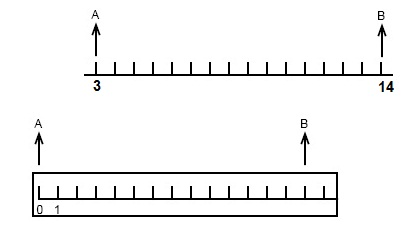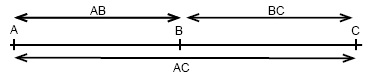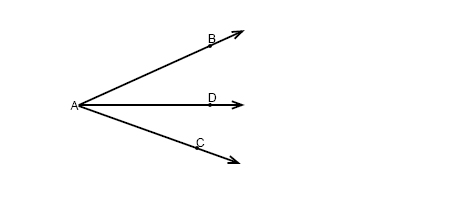# Proofs using algebra

A two column proof is a method to prove statements using properties that justify each step. The properties are called reasons. All reasons used have been showed in previously algebra courses. We will in the following video lesson show how to prove that x=-½ using the two column proof method.

The ruler postulate tells us that two points on a line can be paired with real numbers so that, given any two points A and B, A is zero and B is a positive real number.Further on the segment addition postulate tells us that if a point B is somewhere between point A and point C then AB + BC = AC.similar relationship is the angle addition postulate. If we have the following angls:$then \; m\angle BAD+m\angle DAC=m\angle BAC$

## Video lesson

Solve the following algebraic equation using logic

$2\left (x+\frac{1}{2} \right )=0$